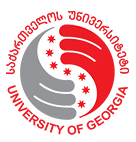## სამეცნიერო კვლევითი ინსტიტუტიკვლევითი პროექტის აღწერა
 პროექტის დასახელება: ფსევდოდიფერენციალური განტოლებები ლის ჯგუფებზე და გამოყენებები მოკლე აღწერა: ხელმძღვანელი: დუდუჩავა როლანდ მონაწილეები: საკითხის აქტუალობა: Many Lie groups are known, but only for some of them, specific models of pseudodifferential equations have been investigated. Recently in  it was discovered that the interval G = (−1, 1) and the cube Q = (−1, 1)n are also Lie groups (see the definition of the group operation below). Then, also in  were computed the corresponding Haar measure, the dual group, the Fourier transform and its inverse (see the next section for the details) for this setting. Then, it turned out that some celebrated equations as the introduced by Prandtl (aerodynamic equation), Tricomi, and Lavrentjev-Bitsadze –emerging from solutions of boundary value problems (BVP) for partial differential equations (PDEs) of mixed type– as well as the Laplace equation on the hemisphere, can be written in the convolution form. Then, in  were discovered explicit formulae for the precise solutions of these equations, while the methods developed prior to this work only allowed the investigation of Fredholm properties and numerical solutions of them, leaving open several questions for the spectral theory of these operators. This investigation wants to study differential, integro-differential, convolution, and more general pseudodifferential equations on different Lie groups. Our approach could give a powerful tool for studying mathematical models in different areas of pure and applied sciences. მოსალოდნელი სიახლე: Main objective of the present research plan is to investigate new classes of pseudodifferential equations on Lie groups (including integral and ordinary /partial differential equations as subclasses) and finding new applications for them in problems of Mathematical Physics, as well as in Practical problems. Very was added successful examples of such equations are the convolution on the Euclidean space Rn (including Wiener-Hopf equations), on the half axes R+ (Mellin convolutions) and discrete convolutions on the lattice of integers Zn (including Toeplitz equations). Recently it was understood that these clas-ses of equations belong to convolutions on Lie groups Rn, R+ and Zn and was added new class-convolutions on the Lie group G=(-1,1), where the group operation is defined as follows (x+y)/(1+xy). Was investigated corresponding convolution equations from the Mathematical Physics equations from the Mathematical Physics equations (criteria of unique solvability) and, using the obtained results, were solved such celebrated equations from the Mathemati-cal Physics as Prandtl, Tricomi and Lavrentjev-Bitsadze equations. The objectives of the project are: A. Study other equations of Mathematical Physics; B. Study convolutions on other Lie groups interesting in applications. სავარაუდო გეგმა: დონორი: ევროკავშირი გრანტი: სკოლა/დეპარტამენტი: დაწყება: 2023-04-21 დასრულება: 2023-07-17 მიზნობრიობა: სამეცნიერო სტატუსი: დასრულებული შედეგი: დაფინანსდა პროექტის ღირებულება: პროექტის პირველადი ხარჯთაღრიცხვა: პროექტის საბოლოო ხარჯთაღრიცხვა: დონორისგან მიღებული თანხა: თანადამფინანსებელი: თანამშრომლობა/პარტნიორობა: პუბლიკაცია: 3

2 55 22 22
კოსტავას 77ა